CiteScore 2.14
More on impact ›

# Frontiers in Built Environment## Original Research ARTICLE

Front. Built Environ., 22 August 2017 | https://doi.org/10.3389/fbuil.2017.00051

# Modal–Physical Hybrid System Identification of High-rise Building via Subspace and Inverse-Mode Methods

• Department of Architecture and Architectural Engineering, Graduate School of Engineering, Kyoto University, Kyoto, Japan

A system identification (SI) problem of high-rise buildings is investigated under restricted data environments. The shear and bending stiffnesses of a shear-bending model (SB model) representing the high-rise buildings are identified via the smart combination of the subspace and inverse-mode methods. Since the shear and bending stiffnesses of the SB model can be identified in the inverse-mode method by using the lowest mode of horizontal displacements and floor rotation angles, the lowest mode of the objective building is identified first by using the subspace method. Identification of the lowest mode is performed by using the amplitude of transfer functions derived in the subspace method. Considering the resolution in measuring the floor rotation angles in lower stories, floor rotation angles in most stories are predicted from the floor rotation angle at the top floor. An empirical equation of floor rotation angles is proposed by investigating those for various building models. From the viewpoint of application of the present SI method to practical situations, a non-simultaneous measurement system is also proposed. In order to investigate the reliability and accuracy of the proposed SI method, a 10-story building frame subjected to micro-tremor is examined.

## Introduction

In response to the rapid advancement in monitoring techniques, the research in the field of structural health monitoring (SHM) has been accelerated (Housner et al., 1997; Boller et al., 2009; Takewaki et al., 2011). Various techniques are used in SHM, and the system identification (SI) methodologies play a key role in SHM. It is understood that the modal-parameter SI and the physical-parameter SI are two major branches in the field of SI. Great concern has been concentrated on the modal-parameter SI (Hart and Yao, 1977; Agbabian et al., 1991; Shinozuka and Ghanem, 1995; Nagarajaiah and Basu, 2009; Takewaki and Nakamura, 2010), which can provide overall mechanical properties of a structural system and has a stable characteristic. For example, the subspace method is known as one of SI algorithms for the modal-parameter SI to identify input–state–output systems in terms of the parametrical system matrices (Van Overschee and De Moor, 1994; Peeters et al., 1997; Huang and Lin, 2001). On the other hand, the physical-parameter SI has another merit in the sense that the physical parameters, e.g., stiffness and/or damping, can be obtained directly, and this is quite effective for the damage detection. Although the physical-parameter SI is preferred in SHM, its advancement is restricted due to the strict requirement of multiple measurements or the necessity of complicated manipulation (Hart and Yao, 1977; Udwadia et al., 1978; Takewaki and Nakamura, 2000; Brownjohn, 2003; Takewaki and Nakamura, 2005; Nagarajaiah and Basu, 2009; Takewaki et al., 2011; Zhang and Johnson, 2013a,b; Johnson and Wojtkiewicz, 2014; Wojtkiewicz and Johnson, 2014).

In the field of physical-parameter SI, Takewaki and Nakamura (2000) developed a smart identification method and introduced a unique SI formulation based on the pioneering work devised by Udwadia et al. (1978). In the method due to Udwadia et al. (1978), the stiffness and damping coefficient at a given story of a shear building model (S model) can be identified directly from the floor acceleration records just above and below the target story using the so-called identification function. However, in the SI method proposed by Takewaki and Nakamura (2000, 2005), there exists an issue to be overcome in applying to actual data, e.g., micro-tremors, due to the small signal/noise (SN) ratio in the low frequency range. Furthermore, an S model is not necessarily a suitable model of high-rise buildings with large aspect ratios due to the influence of building overall bending deformation. The former problem has been a major and most difficult problem in the field of the physical-parameter SI where the limit value evaluation of the transfer function for ω → 0 is needed. The Auto-Regressive with eXogenous (ARX) model with constraints on the ARX parameters has been introduced by Maeda et al. (2011), Kuwabara et al. (2013), and Minami et al. (2013) to avoid the difficulty caused by noise. By applying the ARX model to transfer functions, the difficulty in the evaluation of limit value for small SN-ratio data has been overcome (Fujita et al., 2015). On the other hand, the latter problem has been tackled by expanding the SI algorithm to the shear-bending model (SB model) (Fujita et al., 2013; Minami et al., 2013; Fujita and Takewaki, 2016). However, there exists an unstable phenomenon in identifying the bending stiffness due to the low sensitivity of bending stiffnesses on natural frequencies and/or responses.

In order to develop a hybrid method of the modal-parameter SI and the physical-parameter SI, some researchers proposed a reliable SI method in which the physical parameters are identified from the pre-identified modal parameters (Hjelmstad et al., 1995). However, it needs a deeper consideration of the relation between the physical parameters and the modal parameters together with the detailed theoretical investigation on inverse problem formulation (Hjelmstad, 1996).

In this paper, an SI problem of high-rise buildings is investigated. The shear and bending stiffnesses of an SB model are identified via the subspace and inverse-mode methods. Since the inverse-mode method can provide the shear and bending stiffnesses of the SB model directly from the lowest mode of horizontal displacements and floor rotation angles, the lowest mode of the objective building is identified first by using the subspace method. Identification of the lowest mode is performed by using the amplitude of transfer functions derived in the subspace method (Katayama, 2005). In this paper, the N4SID method is used to derive the transfer function of both horizontal acceleration and floor rotational acceleration of the objective building. By introducing the identification of the lowest-mode shape in the intermediate process and using the inverse-mode formulation, the unstable phenomenon in the identification of the bending stiffness due to the low sensitivity of bending stiffnesses on natural frequencies and/or responses is avoided. Furthermore, considering the resolution in measuring the floor rotation angles in lower stories, floor rotation angles in most stories are predicted from the floor rotation angle at the top floor. An empirical equation of floor rotation angles is proposed by investigating those for various building models. From the viewpoint of the application of the present SI method to practical situations, a non-simultaneous measurement system is also proposed. In order to investigate the reliability and accuracy of the proposed SI method, a 10-story building frame subjected to micro-tremor is examined. Furthermore, the proposed SI method is also applied to the actual record data in the scaled experimental model.

## Stiffness Identification Using Subspace Method and Inverse-Mode Method

In this section, a new stiffness identification method for an SB model is presented using the subspace method and the inverse-mode method. The SB model is considered to be effective in the SI of high-rise buildings because the influence of bending deformation on the structural response, e.g., inter-story drifts, can be estimated appropriately. Figure 1 shows an N-story SB model where the shear and rotational springs are linked as a series type. The number of degrees of freedom in this SB model is 2 N, i.e., N for horizontal responses and N for rotational responses. In the previous researches on the SI method using the SB model (Minami et al., 2013; Fujita and Takewaki, 2016), only the horizontal accelerations observed in the numerical/experimental results were used to identify both shear and bending stiffnesses. Since the measurable data are limited in such identification, it is necessary to apply some optimization algorithms to minimize the error in the identification of modal parameters, i.e., natural frequencies. Therefore, it was needed to investigate the result of identified stiffnesses due to the stability problem caused by the optimization algorithm, e.g., initial value dependency. On the other hand, in this paper, the floor rotational response is also used in the SI.

FIGURE 1

The equations of motion for the SB model subjected to the ground acceleration $u¨g(t)$ are described by

$Mu¨(t)+Cu˙(t)+Ku(t)=−Mru¨g(t)$

where M, C, and K denote the mass, damping, and stiffness matrices of the SB model, and r is the influence coefficient vector defined by {1,…,1,0,…,0}. In addition, u(t) denotes a set of displacements defined by

$u(t)=u1(t),u2(t),...,uN(t)|θ1(t),θ2(t),⋯,θN(t)T$

where uj(t) and θj(t) are the floor horizontal displacement and floor rotation angle of the SB model.

### Estimation of Transfer Function Using Subspace Method

The shear and bending stiffnesses of the SB model can be identified by the inverse-mode method, which will be explained in the following section. In order to apply this inverse-mode method, the lowest-mode shape of all degrees of freedom including horizontal displacements and rotation angles of the SB model is required. In this section, the estimation method of the lowest-mode shape of the SB model is proposed using the subspace method based on the observation of transfer functions.

The subspace method is known as an effective identification algorithm to provide a sequence of the state vector of the state space model described for k = 1, 2, …, Nd as

$x(k+1)=Ax(k)+Bu(k)$
$y(k)=Cx(k)+Du(k)+w(k)$

where u(k), y(k), x(k), w(k), and Nd are the input, output, state vectors, measurement noise caused by the limitation of resolution of sensors, and data length, respectively. Furthermore, A, B, C, and D denote the matrices including the unknown system parameters, which can be derived by the least-squares estimation using the N4SID method. The transfer function G(z) between y(k) and u(k) can be given in terms of A, B, C, and D as

$G(z)=C(zI-A)−1B+D$

where $z=eiωT0$. In this paper, the observed ground acceleration, e.g., earthquake ground motions or micro-tremors, is used as the input vector u(t). On the other hand, the observed floor horizontal accelerations and floor rotational accelerations are used as the output vector y(k).

$u(k)=u¨g(k).$
$y(k)=u1(k),u2(k),⋯,uN(k),θ1(k),θ2(k),⋯,θN(k).$

In this paper, the floor rotational acceleration is evaluated in the numerical simulation using building frame models by dividing the difference of the vertical accelerations, $v¨Rj(t)$ and $v¨Lj(t)$, at the corner columns by the total span length L.

$θ¨j(t)=v¨Rj(t)−v¨Lj(t)L.$

From the viewpoint of the resolution of measurement of floor rotational accelerations in high-rise buildings, the measurement of θi(t) or $θ¨i(t)$ may be difficult especially in lower stories. For this reason, the lowest-mode shape for rotational angles is estimated empirically in a non-simultaneous measurement system.

### Estimation of Lowest-Mode Shape Using Transfer Function Amplitudes

The lowest-mode shape is identified by using the horizontal and rotational transfer functions derived from the subspace method. In this paper, the horizontal and rotational lowest-mode shape is given by the ratio of the transfer function amplitudes at the fundamental natural frequency. The fundamental natural circular frequency ω1 is determined from the frequency that maximizes the amplitude of the transfer function of the horizontal displacement at the top floor. Figure 2 illustrates the summary of the identification of the lowest-mode shape from the transfer functions. The lowest-mode shape of floor horizontal displacements U and floor rotation angles θ can be evaluated as the vector of the obtained transfer function amplitudes {G11), G21), …, G2N1)} at ω = ω1 described by

$U=1ω12U¨,θ=1ω12θ¨$

where $U¨={G1(ω1),G2(ω1),⋯,GN(ω1)}$ and $θ¨={GN+1(ω1),GN+2(ω1),⋯,G2N(ω1)}$. These are derived from the transfer functions as shown in Figure 2.

FIGURE 2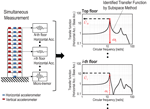Figure 2. Schematic diagram of lowest-mode shape identification based on transfer functions.

Although the simultaneous measurement of the horizontal and floor rotational accelerations is recommended in the proposed SI method, the SI based on the non-simultaneous measurement system, e.g., the relocation of sensors in the measurement system under micro-tremors, is also investigated for the practical application purpose. The non-simultaneous measurement system has a benefit to reduce the initial and maintenance costs due to the small number of sensors. Figure 3 shows the summary of the lowest-mode shape identification in the non-simultaneous measurement system. As shown in Figure 3, in the non-simultaneous measurement system, the top-floor horizontal acceleration and vertical accelerations at the corner columns are needed to stabilize the transfer function evaluation in the subspace method. This is because the amplitude of the transfer function at the i-th floor may be variable due to the difference of utilized micro-tremors in the non-simultaneous measurement system. Although the simultaneous measurement at the top-floor is necessary, it is unnecessary to measure floor accelerations of all stories at the same time. In this paper, we call this system as the non-simultaneous measurement system.

FIGURE 3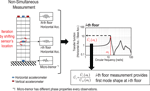Figure 3. Schematic diagram of lowest-mode shape identification in non-simultaneous measurement system.

The lowest-mode shape of the horizontal displacement at the i-th floor is determined by the ratio of the amplitude of the i-th floor transfer function at ω = ω1 to that for the top floor. Finally, the lowest-mode shape of horizontal displacement in the non-simultaneous measurement system can be derived as

$Unon-simul=1ω12U1(1)(ω1)UN(1)(ω1),U2(2)(ω1)UN(2)(ω1),⋯,1$

where the number in the superscript represents the non-simultaneous measurement number index. On the other hand, since the measurement of vertical accelerations in the slab at lower stories is difficult due to the problem of low S/N ratio and resolution, the lowest-mode shape of the floor rotation is necessary to estimate by using an empirical equation. This procedure will be explained in the following section.

### Inverse-Mode Method for Stiffness Identification

The shear and bending stiffnesses ks, kb of the SB model can be derived by substituting the lowest mode of both horizontal displacements and floor rotation angles into the governing equations in the inverse-mode method (Takewaki and Nakamura, 1993; Takewaki, 1999). Figure 4 illustrates the scheme of the inverse-mode method. In the inverse-mode method using the lowest mode of both floor horizontal displacements and rotation angles, these stiffnesses can be determined by

$ks1,ks2,⋯,ksN,kb1,kb2,⋯,kbNT=S−1F$

where

$S=s1−s2000s2−s3⋱0sN00−H1s1ϕ1−ϕ20−H2s2ϕ2−ϕ3⋱⋱−HNsNϕN,F=ω12m1u1⋮mNuNI1θ1⋮INθN$
$sj=uj−Hjθj(j=1)uj−uj−1−Hjθj(j=2,⋯,N),ϕj=θ1(j=1)θj−θj−1(j=2,⋯,N).$
FIGURE 4

As seen in Figure 4, sj and ϕj are the inter-story shear deformation and the inter-floor rotation angle. In addition, Hj is the story height of the j-th story.

## Empirical Equation of Floor Rotation Angle in Lowest-Mode Shape

Since the amplitude of floor vertical acceleration, especially in the lower stories, is relatively small and easily affected by the measurement noise, the reliable measurement of the floor rotation angle may be difficult from the practical point of view. The lowest-mode shape of the floor rotation angle is therefore derived here empirically by using only the floor rotational angle observed at the top floor. In this section, the empirical equation is proposed by comparing various mode shapes of plane building frames.

### Building Frames

In this section, 5-, 10-, and 20-story plane steel building frames are used to investigate the influence of various structural properties, e.g., number of stories, number of spans, story-wise stiffness distribution, on the lowest-mode shape of floor rotation angle. The reference lowest-mode shape of the floor rotation angle of those building models can be derived by using the simultaneously measured floor rotation angle obtained in the abovementioned lowest-mode shape identification based on the subspace method.

Table 1 shows the cross-sectional list of columns and beams, and Table 2 presents the properties of building models. The structural damping (damping ratio: 0.02) is given as the stiffness proportional damping. In 5-story building models, models 1, 2, and 3 are used to investigate the influence of the number of spans. In addition, the effect of the difference of the stiffness distribution is compared in models 1, 4, and 5. Since the stiffness distribution affects the lowest-mode shape of the floor rotation angle, models 6, 7, 8 and models 9, 10 are examined for 10-story and 20-story building models where the stiffness distributions are different.

TABLE 1
TABLE 2

### Lowest-Mode Shape of Floor Rotation Angle

Figure 5 shows the comparison of the identified lowest-mode shape of floor rotation angles for 5-, 10-, and 20-story building models listed in Table 2. In this figure, the empirically estimated lowest-mode shapes are also shown. It can be observed from Figure 5A that the mode shapes of models 1, 2, and 3 may not be affected by the number of spans and span length. Furthermore, from the parametric analysis for various building frames except those in Table 2, it has also been confirmed that the aspect ratio and the distribution of story stiffness may slightly affect the mode shape of floor rotation angle. Based on these results, in order to obtain the empirical equation for the lowest-mode floor rotation angle, the amplitude of floor rotation angle θj at the j-th story is assumed to be given by using the just upper story floor rotation angle θj + 1 by

$θj=θj+1−A⋅θj+1(N−j)2$

where A is a shape coefficient. In Eq. 13, the floor rotation angle in the top story can be given by the obtained floor vertical accelerations and Eq. 7. The coefficients A in Eq. 13 is needed to be modified by the number of stories. From the results for various building frames in Table 2 and other models, the coefficients A can be derived by the least-squares approach that can be categorized by the number of stories as

$A=0.0250(for5-storybuilding)0.0040(for10-storybuilding)0.0012(for15-storybuilding)0.0005(for20-storybuilding).$
FIGURE 5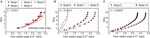Figure 5. Comparison of identified lowest-mode shape with empirically estimated one, (A) 5-story building, (B) 10-story building, (C) 20-story building.

The shape coefficient A can be derived in an exponential form as

$A(N)=0.2332×e−0.49N+0.01053×e−0.1537N$

where N is the number of stories of the objective building.

## Numerical Examples

### Objective Buildings

Consider a 10-story plane building frame. The story height Hj, story mass mj, and rotary inertia Jj are constant in all stories as 4.0 m, 120,000 kg, 1,620,000 kgm2, respectively. The number of spans is four, and the common span length is 3.0 m. The stiffness proportional damping ratio is 0.02. Table 3 shows the cross-sectional list. The output time history data, i.e., horizontal and vertical accelerations at the corner column nodes, are calculated by the response analysis software, SNAP (2015).

TABLE 3

### Micro-Tremor

The micro-tremor recorded in the Disaster Prevention Research Institute of Kyoto University is used as the input ground acceleration. Figure 6 shows an example of the time history and the acceleration response spectrum of the micro-tremor. The measurement duration of the micro-tremor is longer than 5 min. The sampling frequency is 100 Hz. In the following numerical examples, the band-limited white noise (from 0.1 to 20 Hz) is added to the output data, i.e., horizontal and rotational acceleration, independently at each story. The root-mean-square (RMS) of the noise is determined for the horizontal acceleration and rotation angle, respectively. These amplitudes of the noise are constant at all stories and given so as to make the 10 or 20% RMS of noise to that of the responses at the first story.

FIGURE 6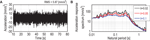Figure 6. Input ground acceleration (micro-tremor). (A) Time history, (B) acceleration response spectrum (h: damping ratio).

### Stiffness Identification of SB Model in Simultaneous Measurement

First of all, in order to investigate the accuracy of the stiffness identification algorithm, shear and bending stiffnesses of the SB model are identified by using all floor horizontal and rotational responses measured simultaneously under the same input. Figure 7 shows the transfer functions for horizontal accelerations without noise derived by the subspace method. From these transfer functions including floor rotation angles, the lowest-mode shape can be derived from the amplitude of them as explained in Eq. 8. After obtaining the lowest-mode shape, the shear and bending stiffnesses of the SB model can be determined directly without any additional operation. Figure 8 shows the comparison of the identified stiffnesses, including the case considering noise-biased data, with the reference stiffnesses of the objective building frame and the shear stiffness identified as the S model. For identifying as the S model, the inverse-mode method in Eq. 10 can be reduced to N × N matrices. The reference stiffnesses in Figure 8 can be derived from the pushover analysis result using the object building frame. Table 4 shows the comparison of the identified natural periods with the reference ones. As shown in Table 4, it can be observed that the SB model is more appropriate to represent high-rise building frames than the S model.

FIGURE 7
FIGURE 8
TABLE 4

### Verification of Identified SB Model in Non-Simultaneous Measurement

In this section, the identification accuracy in shear and bending stiffness of the SB model is investigated in the case of non-simultaneous measurement. Furthermore, the influence of noise added to the output data on the identification accuracy is also studied. As explained before, in the non-simultaneous measurement, the horizontal and rotational accelerations at the top story are required simultaneously in addition to the horizontal accelerations at each objective story and at the base. This is because the lowest-mode shape can be predicted more accurately by using the transfer function’s amplitude at the i-th floor normalized by that of the top story. Figures 9A,B show the identified participation vector of both horizontal displacement and floor rotation angle derived from the subspace method. Furthermore, the shear and bending stiffnesses in the non-simultaneous measurement with noise are shown in Figures 9C,D. Table 5 shows the error (ratio) of natural periods of the identified SB models. From Figure 9, the identified bending stiffnesses in the non-simultaneous measurement are a little different from the reference values even in the case without noise. This difference may be caused by the influence of the reliability of the empirical equation on the lowest-mode shape of floor rotation angle.

FIGURE 9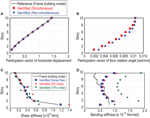Figure 9. Identified shear stiffness and bending stiffness in non-simultaneous measurement. (A) Identified participation vector of horizontal displacement, (B) identified participation vector of floor rotation angle, (C) identified shear stiffness, and (D) identified bending stiffness.

TABLE 5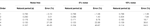Table 5. Error ratio of identified natural periods in non-simultaneous measurement with/without noise.

In practical building frames, the lowest-mode shape of the inter-floor rotation angle is non-monotonic due to irregular column section distributions. In order to estimate the bending stiffness of the SB model more accurately, it may be necessary to consider these factors in the empirical equation. However, as summarized in Table 5, the natural periods of the identified SB model in non-simultaneous measurement using the output data with noise can be obtained in a reliable manner. This may result from several facts. First, the bending stiffness of the SB model has slight influence on the natural periods. Second, the shear and bending stiffnesses of the SB model are influenced by each other. Therefore, as seen in Figure 9, the shear stiffness of the SB model varies in the case of 5 and 10% noise. Figure 10 shows the comparison of the top-story acceleration as the seismic response of the identified SB model with that of the building frame model under El Centro NS (1940). As shown in Figure 10, the seismic response of the frame building model can be predicted in a reliable manner by the SB model identified using the noise-biased output data. Although the required accuracy in the prediction of the seismic response by using the identified physical model may vary depending on the situation, it may be concluded that minimizing the identification error on the modal properties, i.e., natural frequencies including higher modes, is important for this purpose.

FIGURE 10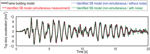Figure 10. Comparison of top-story horizontal accelerations in identified shear-bending model (SB model) under El Centro NS (1940).

## Application to Stiffness Identification in Experimental Scaled Structure

In this section, the proposed SI method is applied to actual data measured in a scaled structure. A similar experiment was conducted by Fujita et al. (2013) to show the applicability of the previously proposed SI method. The accuracy and reliability of the proposed SI method in the non-simultaneous measurement are investigated by using micro-tremor data.

### Scaled Structure

A scaled structure was designed so that certain amounts of remarkable shear and bending deformations can be measured, and this model has an appropriate ratio of bending deformation to shear deformation considering high-rise buildings. Figure 11 shows a photograph and schematic diagram of the scaled structure; floor plates, column plates, spacer blocks (SUS304), and angle bars supporting column (steel). The objective direction to identify the story stiffness of the scaled structure is x in Figure 11. It is known that the width of the spacer block is correlated with the bending stiffness. In this experimental study, the width of the spacer block at the first story is 20 mm and those at the both second and third stories are 10 mm. In order to prevent the rocking vibration of the scaled structure, the base plate is fixed at the shaking table.

FIGURE 11

The servo-type velocity-meter (VSE-15D, Tokyo Sokushin) was used to simulate the micro-tremor measurement. The resolution of velocity of this velocity meter is 10−4 mm/s. The S/N ratio of this experiment was confirmed to be larger than 60 dB at the base input. Since the mass of this velocity-meter is not negligible compared with that of the floor mass, it was modeled by an additional mass, and the location of the velocity meter was changed so as to simulate the situation of non-simultaneous measurement. Another additional mass was placed both at the first and second floors to reduce the influence of mass variation and shifting of the center of mass. Considering the additional mass, the lumped mass and rotational inertia of each story were given as m1 = 4.02 kg, m2 = 3.72 kg, m3 = 3.05 kg and I1 = 6.30 × 10−3 kgm2, I2 = 5.84 × 10−3 kgm2, I3 = 4.78 × 10−3 kgm2.

Figure 12 shows the time histories of input and output measured data in the non-simultaneous measurement. It can be confirmed that the amplitudes of the measured input micro-tremor are almost the same among these several measurements. It can also be observed that the vertical velocities at the left and right ends of the top story exhibit opposite-phase. From the measured input and output data, the transfer function at each story can be derived by the subspace method. The identified transfer functions of the horizontal and rotational accelerations to the base acceleration are shown in Figure 13. In this figure, the transfer functions of the horizontal acceleration derived by the sweep testing are also shown. As seen in Figure 13, the transfer function identified by the subspace method seems to be derived correctly. It was confirmed that, by applying the subspace method, the transfer functions can be derived appropriately. Finally, the shear and bending stiffnesses of the SB model have been obtained from the identified lowest-mode shape. The comparison of the natural frequencies of the identified SB model with those observed by the sweep-vibration test result is shown in Table 6. Although a slight difference can be seen in the third natural frequency, the SB model does not necessarily provide the actual modal properties (especially higher modes).

FIGURE 12
FIGURE 13
TABLE 6

In order to investigate the accuracy of the identified SB model, the comparison of the time history records for the random input between the simulated and the measured has been conducted in a scaled structure using a shaking table. By using the identified SB model in the numerical simulation, the horizontal displacements and accelerations can be estimated for the specified input motion. The input acceleration is given by the measured data on the shaking table. Figure 14 shows the time history of the absolute acceleration at the top story together with the base input, and Figure 15 presents the story-wise distribution of the maximum acceleration. In this verification, the target time history of ground acceleration on the shaking table is El Centro NS (1940), where the amplitude is controlled to 10% of the original wave (see Figure 14A). In Figure 15, the maximum error ratio is 15.4% in the first story. In order to predict the acceleration responses reliably, the modal properties including higher modes are needed to be identified correctly. As for the merit of the proposed SB model compared to the S model, it has been made clear before (Minami et al., 2013) that the S model cannot simulate the acceleration in an accurate manner because the S model cannot express the higher natural frequencies of the actual structure accurately. However, it is also true that the accurate simulation of acceleration is difficult even by the SB model due to high sensitivity of acceleration to structural and input motion parameters. Since the present paper uses the modal–physical hybrid method for the SB model different from the previous method (Minami et al., 2013) using a direct physical-parameter SI, the stiffness parameters can be identified in a more stable manner. It may be concluded that the SB model has been identified appropriately by the proposed method and can be used to predict the response of the objective structure even under noise-biased environment.

FIGURE 14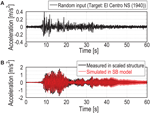Figure 14. Comparison of time history response of scaled structure with identified shear-bending model (SB model). (A) Base input on shaking table (B) top-floor horizontal acceleration.

FIGURE 15

## Conclusion

This paper presented a stiffness identification method using an SB model for high-rise buildings based on the subspace method and the inverse-mode method. The subspace method was applied to estimate the lowest-mode shape of both floor horizontal displacements and floor rotation angles. By using the identified lowest-mode shape, the shear and bending stiffnesses of the SB model were directly determined by the inverse-mode method. The following conclusions were derived.

(1) A new stiffness identification method using an SB model for high-rise buildings has been proposed via the subspace method and the inverse-mode method. The lowest-mode shape of horizontal displacement and floor rotation angle has been estimated from the transfer functions derived by the subspace method. Then the shear and bending stiffnesses have been determined from the identified lowest-mode shape of both horizontal and rotational degrees of freedom by using the inverse-mode method. It has been confirmed that the lowest-mode shape can be estimated in a reliable manner from the amplitudes of the transfer functions at the fundamental natural frequency.

(2) Since the measurement of floor rotation angles in high-rise buildings is difficult due to the resolution problem in lower stories, an empirical equation of floor rotation angles has been proposed in which only the top-floor rotation angle is required. This empirical equation has been determined by the numerical simulations of building frames considering various structural parameters such as aspect ratio, number of spans, span length, and stiffness distribution.

(3) In order to apply the present stiffness identification method to the non-simultaneous measurement system, a modification in the lowest-mode shape’s identification has been introduced. In the non-simultaneous measurement system, it has been confirmed that the top-floor horizontal responses, i.e., horizontal and vertical acceleration or velocity, are needed to be measured in addition to the target story and the base. This is because the identified transfer function using the subspace method can be stabilized by employing the top-floor responses simultaneously. Furthermore, the lowest-mode shape can be identified more accurately by the transfer function’s amplitude at the specified story normalized by that of the top story. In this modified identification method for the lowest-mode shape, it has been verified that the lowest-mode shape of horizontal and rotational responses can be derived appropriately even when considering noise.

(4) As a verification of the present stiffness identification method, a numerical simulation and a scaled experiment have been examined for plane building frames. From these verifications, it has been confirmed that the proposed identification method can be used as an SI method more reliable than the previous methods.

The S/N ratio of the records may be a key issue, and wind disturbances may affect the accuracy of the proposed method. Although numerical investigations and scaled-model experiments were conducted in the present paper, application of the proposed method to actual buildings may be necessary.

## Author Contributions

KF formulated the problem, conducted the computation, and wrote the paper. YF formulated the problem and conducted the computation. IT supervised the research and wrote the paper.

## Conflict of Interest Statement

The authors declare that the research was conducted in the absence of any commercial or financial relationships that could be construed as a potential conflict of interest.

## Funding

Part of the present work is supported by the Grant-in-Aid for Scientific Research (KAKENHI) of Japan Society for the Promotion of Science (No. 16K18184, 15H04079).

## References

Agbabian, M. S., Masri, S. F., Miller, R. K., and Caughey, T. K. (1991). System identification approach to detection of structural changes. J. Eng. Mech. 117, 370–390. doi: 10.1061/(ASCE)0733-9399(1991)117:2(370)

Boller, C., Chang, F.-K., and Fujino, Y. (eds) (2009). Encyclopedia of Structural Control and Health Monitoring, Vol. 1-5. Chichester: Wiley.

Brownjohn, J. M. W. (2003). Ambient vibration studies for system identification of tall building. Earth Eng. Struct. Dyn. 32, 71–95. doi:10.1002/eqe.215

Fujita, K., Ikeda, A., Shirono, M., and Takewaki, I. (2013). “System identification of high-rise buildings using shear-bending model and ARX model: experimental investigation,” in Proc. of ICEAS13 in ASEM13 (Jeju, Korea), 2803–2815.

Fujita, K., Ikeda, A., and Takewaki, I. (2015). Application of story-wise shear building identification method to actual ambient vibration. Front. Built Environ. 1:Article 2. doi:10.3389/fbuil.2015.00002

Fujita, K., and Takewaki, I. (2016). Advanced system identification for high-rise building using shear-bending model. Front. Built Environ. 2:Article 29. doi:10.3389/fbuil.2016.00029

Hart, G. C., and Yao, J. T. P. (1977). System identification in structural dynamics. J. Eng. Mech. Div. 103, 1089–1104.

Hjelmstad, K. D. (1996). On the uniqueness of modal parameter estimation. J. Sound Vib. 192, 581–598. doi:10.1006/jsvi.1996.0205

Hjelmstad, K. D., Banan, M. O. R., and Banan, M. A. R. (1995). On building finite element models of structures from modal response. Earthquake Eng. Struct. Dyn. 24, 53–67. doi:10.1002/eqe.4290240105

Housner, G., Bergman, L. A., Caughey, T. K., and Chassiakos, A. G. (1997). Special issue, structural control: past, present, and future. J. Eng. Mech. 123, 897–971. doi:10.1061/(ASCE)0733-9399(1997)123:9(897)

Huang, C. S., and Lin, H. L. (2001). Modal identification of structures from ambient vibration, free vibration, and seismic response data via a subspace approach. Earthquake Eng. Struct. Dyn. 30, 1857–1878. doi:10.1002/eqe.98

Johnson, E. A., and Wojtkiewicz, S. F. (2014). Efficient sensitivity analysis of structures with local modifications. II: transfer functions and spectral densities. J. Eng. Mech. 140. doi:10.1061/(ASCE)EM.1943-7889.0000769

Katayama, T. (2005). Subspace Methods for System Identification. London: Springer-Verlag.

Kuwabara, M., Yoshitomi, S., and Takewaki, I. (2013). A new approach to system identification and damage detection of high-rise buildings. Struct. Control Health Monit. 20, 703–727. doi:10.1002/stc.1486

Maeda, T., Yoshitomi, S., and Takewaki, I. (2011). Stiffness-damping identification of buildings using limited earthquake records and ARX model. J. Struct. Construct. Eng. 666, 1415–1423. doi:10.3130/aijs.76.1415

Minami, Y., Yoshitomi, S., and Takewaki, I. (2013). System identification of super high-rise buildings using limited vibration data during the 2011 Tohoku (Japan) earthquake. Struct. Control Health Monit. 20, 1317–1338. doi:10.1002/stc.1537

Nagarajaiah, S., and Basu, B. (2009). Output only modal identification and structural damage detection using time frequency & wavelet techniques. Earthquake Eng. Eng. Vib. 8, 583–605. doi:10.1007/s11803-009-9120-6

Peeters, B., De Roeck, G., Pollet, T., and Schueremenas, L. (1997). “Stochastic subspace techniques applied to parameter identification of civil engineering structures,” in New Advances in Modal Synthesis of Large Structure, ed. L. Jezequel (Rotterdam: A.A. Balkema), 145–156.

Shinozuka, M., and Ghanem, R. (1995). Structural-system identification II: experimental verification. J. Eng. Mech. 121, 265–273. doi:10.1061/(ASCE)0733-9399(1995)121:2(265)

SNAP. (2015). Structure Non-Linear Analysis Program (Ver.7). Tokyo: Kozo System Inc.

Takewaki, I. (1999). Inverse stiffness design of shear-flexural building models including soil-structure interaction. Eng. Struct. 21, 1045–1054. doi:10.1016/S0141-0296(98)00065-0

Takewaki, I., and Nakamura, M. (1993). Seismic-deformation constrained design of elastically supported shear-flexural building models via hybrid inverse formulation. J. Struct. Eng. 39, 105–118.

Takewaki, I., and Nakamura, M. (2000). Stiffness-damping simultaneous identification using limited earthquake records. Earth. Eng. Struct. Dyn. 29, 1219–1238. doi:10.1002/1096-9845(200008)29:8<1219::AID-EQE968>3.0.CO;2-X

Takewaki, I., and Nakamura, M. (2005). Stiffness-damping simultaneous identification under limited observation. J. Eng. Mech. 131, 1027–1035. doi:10.1061/(ASCE)0733-9399(2005)131:10(1027)

Takewaki, I., and Nakamura, M. (2010). Temporal variation of modal properties of a base-isolated building during an earthquake. J. Zhejiang Univ. Sci. A 11, 1–8. doi:10.1631/jzus.A0900462

Takewaki, I., Nakamura, M., and Yoshitomi, S. (2011). System Identification for Structural Health Monitoring. UK: WIT Press.

Udwadia, F. E., Sharma, D. K., and Shah, P. C. (1978). Uniqueness of damping and stiffness distributions in the identification of soil and structural systems. J. Appl. Mech. 45, 181–187. doi:10.1115/1.3424224

Van Overschee, P., and De Moor, B. (1994). N4SID: subspace algorithms for the identification of combined deterministic-stochastic systems. Automatica 30, 75–93. doi:10.1016/0005-1098(94)90230-5

Wojtkiewicz, S. F., and Johnson, E. A. (2014). Efficient sensitivity analysis of structures with local modifications. I: time domain responses. J. Eng. Mech. 140. doi:10.1061/(ASCE)EM.1943-7889.0000768

Zhang, D., and Johnson, E. (2013a). Substructure identification for shear structures I: substructure identification method. Struct. Control Health Monit. 20, 804–820. doi:10.1002/stc.1497

Zhang, D., and Johnson, E. (2013b). Substructure identification for shear structures with nonstationary structural responses. J. Eng. Mech. 139, 1769–1779. doi:10.1061/(ASCE)EM.1943-7889.0000626

Keywords: system identification, subspace method, inverse-mode method, shear-bending model, high-rise building

Citation: Fujita K, Fujimori Y and Takewaki I (2017) Modal–Physical Hybrid System Identification of High-rise Building via Subspace and Inverse-Mode Methods. Front. Built Environ. 3:51. doi: 10.3389/fbuil.2017.00051

Received: 26 May 2017; Accepted: 02 August 2017;
Published: 22 August 2017

Edited by:

Solomon Tesfamariam, University of British Columbia, Canada

Reviewed by:

Masayuki Kohiyama, Keio University, Japan
Hossein Mostafaei, FM Global, United States

Copyright: © 2017 Fujita, Fujimori and Takewaki. This is an open-access article distributed under the terms of the Creative Commons Attribution License (CC BY). The use, distribution or reproduction in other forums is permitted, provided the original author(s) or licensor are credited and that the original publication in this journal is cited, in accordance with accepted academic practice. No use, distribution or reproduction is permitted which does not comply with these terms.

*Correspondence: Izuru Takewaki, takewaki@archi.kyoto-u.ac.jp